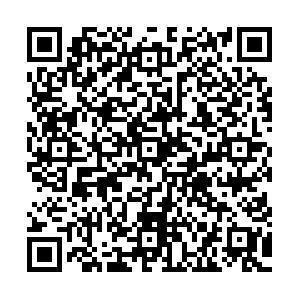# QCD phase diagram at finite isospin chemical potential and temperature in an IR-improved soft-wall AdS/QCD model• We study the phase transition between the pion condensed phase and normal phase, as well as chiral phase transition in a two flavor (${\cal{N}}_f=2$) IR- improved soft-wall AdS/QCD model at finite isospin chemical potential $\mu_I$and temperature T. By self-consistently solving the equations of motion, we obtain the phase diagram in the plane of $\mu_I$and T. The pion condensation appears together with a massless Nambu-Goldstone boson $m_{\pi_1}(T_c, \mu_I^c)=0$, which is very likely to be a second-order phase transition with mean-field critical exponents in the small $\mu_I$region. When $T=0$, the critical isospin chemical potential approximates to vacuum pion mass $\mu_I^c \approx m_0$. The pion condensed phase exists in an arched area, and the boundary of the chiral crossover intersects the pion condensed phase at a tri-critical point. Qualitatively, the results are in good agreement with previous studies on lattice simulations and model calculations.
••Get Citation
Xuanmin Cao, Hui Liu, Danning Li and Guanning Ou. QCD phase diagram at finite isospin chemical potential and temperature in an IR-improved soft-wall AdS/QCD model[J]. Chinese Physics C. doi: 10.1088/1674-1137/44/8/083106
Xuanmin Cao, Hui Liu, Danning Li and Guanning Ou. QCD phase diagram at finite isospin chemical potential and temperature in an IR-improved soft-wall AdS/QCD model[J]. Chinese Physics C.Milestone
Article Metric

Article Views(348)
Cited by(0)
Policy on re-use
To reuse of Open Access content published by CPC, for content published under the terms of the Creative Commons Attribution 3.0 license (“CC CY”), the users don’t need to request permission to copy, distribute and display the final published version of the article and to create derivative works, subject to appropriate attribution.
###### 通讯作者: 陈斌, bchen63@163.com
• 1.

沈阳化工大学材料科学与工程学院 沈阳 110142

Title:
Email:

## QCD phase diagram at finite isospin chemical potential and temperature in an IR-improved soft-wall AdS/QCD model

###### Corresponding author: Danning Li, lidanning@jnu.edu.cn
• Department of Physics and Siyuan Laboratory, Jinan University, Guangzhou 510632, China

Abstract: We study the phase transition between the pion condensed phase and normal phase, as well as chiral phase transition in a two flavor (${\cal{N}}_f=2$) IR- improved soft-wall AdS/QCD model at finite isospin chemical potential $\mu_I$and temperature T. By self-consistently solving the equations of motion, we obtain the phase diagram in the plane of $\mu_I$and T. The pion condensation appears together with a massless Nambu-Goldstone boson $m_{\pi_1}(T_c, \mu_I^c)=0$, which is very likely to be a second-order phase transition with mean-field critical exponents in the small $\mu_I$region. When $T=0$, the critical isospin chemical potential approximates to vacuum pion mass $\mu_I^c \approx m_0$. The pion condensed phase exists in an arched area, and the boundary of the chiral crossover intersects the pion condensed phase at a tri-critical point. Qualitatively, the results are in good agreement with previous studies on lattice simulations and model calculations.

### HTML2.   IR-improved soft-wall AdS/QCD model with finite ${\mu_I}$3.   QCD phase transition4.   Phase diagram
Reference (92)
PDF查看关注分享

Top

### 目录/DownLoad:  Full-Size Img  PowerPoint